RoundVerts tools not only round vertices ,but also optimize poly object by decreasing or increasing the number of vertices .Usually ,road net is turned from spline shape, the number of vertices in corners maybe insufficient or too many,you often need to keep a balance between number vertices and accuracy.With RoundVerts tools ,you don't worry about the steps in shape-editing stage.

### RoundVerts:

A RoundVerts tool with UI,you can manually input parameters such as the number of vertices and the radius of an Arc .This tool provide 3 methods to control how to round vertices: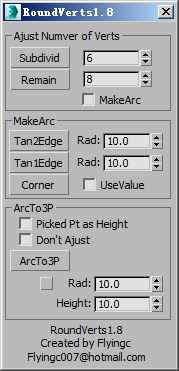RoundVerts

Tan2Edge the arc tangent to two edges.

Tan1Edge the arc of vertices tangent to one edge.

ArcTo3p the arc through three points, two is the positions of two end vertices,one is a point snapped,moving mouse can change arc radius.

Below 3 RoundVerts tools is all one-key, more powerful and very rapid, which use more efficent methods to round verts,can auto figure out radius of arc and how many verts should be remained.

### Auto RoundVerts Tools:ArcVts(3P) | ArcVts(Rad) |ArcVts(TT)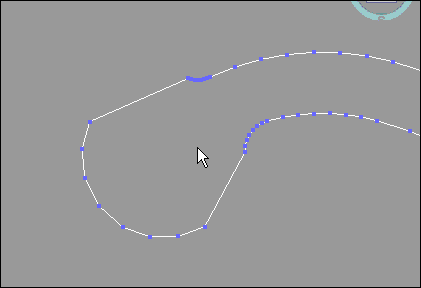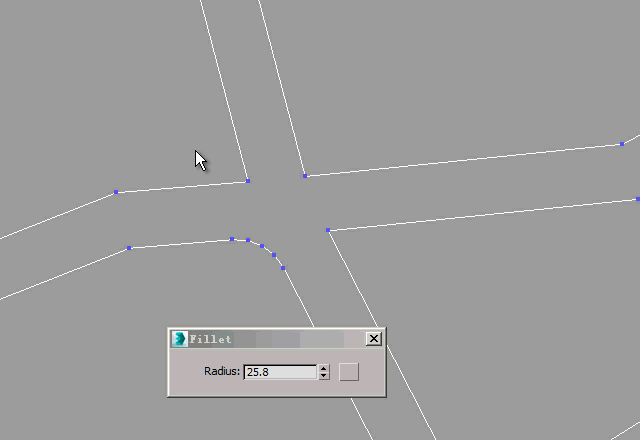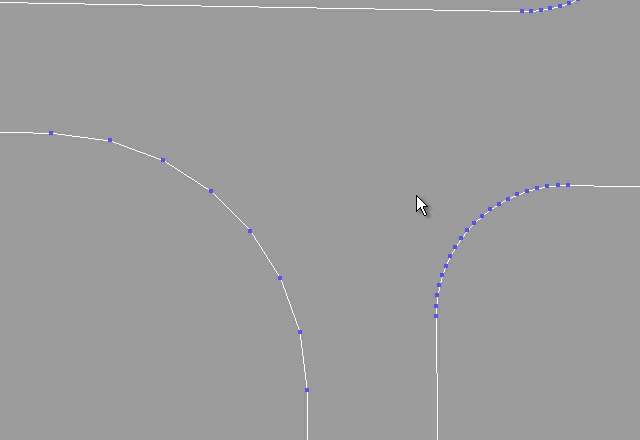ArcVts(3P)Select at least three continuous verts,from which take start vert, end vert,and a random vert to caculate an arc through these 3 verts to round selected vertices.
ArcVts(Rad)Fillet a vertex and set its radius,or select more vertices to round and ajust its radius through a spinner in UI.
ArcVts(TT)Select a continuous vertices(at least 2),take adjacent two edges as tangents to caculate an arc to round.-->

#### How to Control Number of Vertices on an Arc

Number of Vertices on an arc is controled via "SegsVsArc" under "Manager".For arcs with same degree,the bigger the radius ,the more vertices are needed.So I defined a table to describe the mapping relation:Radius versus degree that each segment of Arc occupy,thus the vertices number on an Arc will be gotten via interpolation.
If you need more vertices,you can follow steps blow:

1. import an arc(select an segment of a spline or continous vertices which can form an arc) to get the radius
2. then enter the desired numbers on the arc
3. press "=>Rescale" .
4. Then the change will be applied to Auto RoundVerts tools and Piece poly.
The video below show the operating.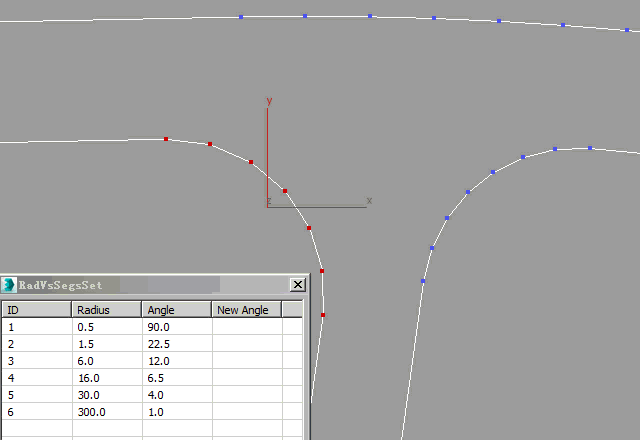Control Number of Vertices